# How to Calculate Cumulative Product in MATLAB

• Last Updated : 18 Oct, 2021

The cumulative product of a sequence is a running products or partial products of a sequence

The cumulative products of the sequence {a,b,c,…}, are a, a*b, a*b*c, ….

Matlab allows us to calculate the cumulative product of a vector, matrix using cumprod() method. Differenct syntaxes of cumprod() method are

• B = cumprod(A)
• B = cumprod(A,dim)
• B = cumsprod(___,direction)
• B = cumprod(___,nanflag)

Now we will discuss the above syntaxes in detail:

### B = cumprod(A)

• The method returns the cumulative product of A starting at the beginning of array A.
• If A is a vector, then it returns the cumulative product of sequence A
• If A is a matrix, then it returns the cumulative product along each column of A.

Example 1:

## Matlab

 `% Input vector``A = 2:8;` `B = cumprod(A);` `% Displays cumulative``% products of A``disp(B)`

Output :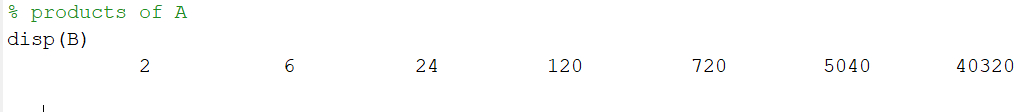Example 2:

## Matlab

 `% Input matrix``A = [1 4 7; 2 5 8; 3 6 9];``disp(``"Matrix :"``)``disp(A)` `B = cumprod(A);``% Display cumulative product of matrix A``disp(``"Cumulative product :"``)``disp(B)`

Output :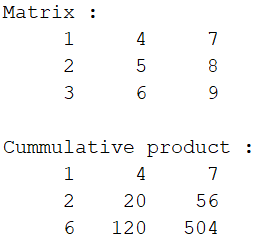### B = cumprod(A,dim)

• Returns the cumulative product of matrix A along with each dim.
• dim takes two values 1 or 2.
• dim = 1, refers to along each column.
• dim = 2, refers along each row.

## Matlab

 `% input matrix``A = [1 3 5; 2 4 6];``disp(``"Matrix :"``)``disp(A)` `% Cumulative product along each``% row from left to right``B = cumprod(A,2);``disp(``"Cumulative product :"``)``disp(B);`

Output :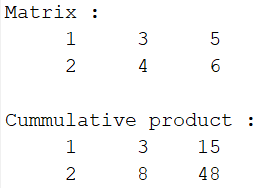### B = cumprod(___,direction)

• Returns the cumulative product of the input vector or matrix in the given direction.
• direction takes two values ‘forward’ or ‘reverse’
• If the direction is ‘reverse’, then calculates cumulative product in reverse i.e if we are considering matrix along each column, then it returns cumulative product starting from bottom to top of each column.

## Matlab

 `% input matrix``A = [1 3 5; 2 4 6];``disp(``"Matrix :"``)``disp(A)` `% Cumulative product of A along each row``% starting from right to left``B = cumprod(A,2,``'reverse'``);``disp(``"Cumulative product :"``)``disp(B)`

Output :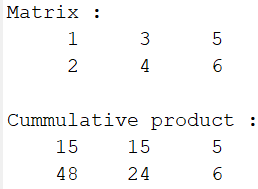### B = cumprod(___,nanflag)

• nanflag value decides whether to include or exclude NaN value of the vector in cumulative product or not.
• nanflag takes two values ‘includenan’ or ‘omitnan’ corresponds to including NaN elements and excluding NaN elements respectively.
• ‘omitNaN’ considers NaN values as 1.

Note: NaN * number = NaN

## Matlab

 `% Input vector``A = [3 5 NaN 9 0 NaN];``disp(``"Vector :"``);``disp(A);` `% Including NaN values``B = cumprod(A,``'includenan'``);``disp(``"Cumulative product Include NaN :"``);``disp(B);` `% Excluding NaN values``B = cumprod(A,``'omitnan'``);``disp(``"Cumulative product Exclude NaN :"``);``disp(B);`

Output :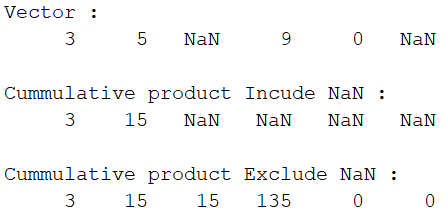My Personal Notes arrow_drop_up